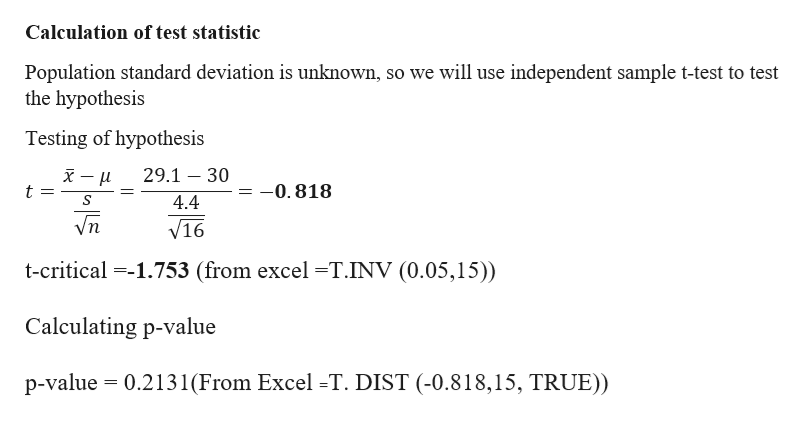# Assume that a simple random sample has been selected from a normally distributed population and test the given claim. Use the p value method and fill in the blanks to address the claim.A manufacturer makes ball bearings that are supposed to have a mean weight of 30g. A retailer suspects that the mean weight is actually less than 30g. The mean weight for a random sample of 16 ball bearings is 29.1g with a standard deviation of 4.4g. At the 0.05 significance level, test the claim that the sample comes from a population with a mean weight less than 30g. Please answer the following with details and the calculator steps:Claim:   __________________________________H0                    H1u0                     xSx                    nt                      pSince p ___________ a, we _______________ the null hypothesis.Final statement:  _____________________________________________

Question

Assume that a simple random sample has been selected from a normally distributed population and test the given claim. Use the p value method and fill in the blanks to address the claim.

A manufacturer makes ball bearings that are supposed to have a mean weight of 30g. A retailer suspects that the mean weight is actually less than 30g. The mean weight for a random sample of 16 ball bearings is 29.1g with a standard deviation of 4.4g. At the 0.05 significance level, test the claim that the sample comes from a population with a mean weight less than 30g.

Claim:   __________________________________

H0                    H1

u0                     x

Sx                    n

t                      p

Since p ___________ a, we _______________ the null hypothesis.

Final statement:  _____________________________________________

check_circleExpert Solution
Step 1

Given data
Hypothesized mean µ= 30
Sample Standard deviation s = 4.4
Sample mean = 29.1
Sample size n = 4.4
Claim - sample comes from a population with a mean weight less than 30g
Formulation of hypothesis
Null Hypothesis∶H_0: μ=30,That is mean weight of ball bearing is 30g
Alternati...help_outlineImage TranscriptioncloseCalculation of test statistic Population standard deviation is unknown, so we will use independent sample t-test to test the hypothesis Testing of hypothesis 29.1 30 -0.818 t = 4.4 V16 t-critical 1.753 (from excel -T.INV (0.05,15)) Calculating p-value 0.2131(From Excel -T. DIST (-0.818,15, TRUE)) p-value fullscreen

### Want to see the full answer?

See Solution

#### Want to see this answer and more?

Solutions are written by subject experts who are available 24/7. Questions are typically answered within 1 hour*

See Solution
*Response times may vary by subject and question
Tagged in

### Statistics